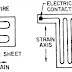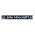A strain gauge is a sensor whose measured electrical resistance varies with changes in strain. Strain is the deformation or displacement of material that results from applied stress. Stress is the force applied to a material, divided by the material’s cross-sectional area.

Working Principle of Strain Gauge Transducer:

The resistance of the wire is proportional to its length and inversely proportional to the diameter (R∝L, R ∝ 1⁄D). If we stretch the wire by an external force, then the length of the wire increases and the diameter reduces, this changes the resistance of the wire.

The force can be measured by measuring this resistance change.

Construction and working of Strain Gauge Transducer:

Strain gauge wires are normally made from the thin constant wire 0.025 mm diameter. It is fitted between the two plastic or ceramic plates. It is stuck to the surface of the system whose force is to be measured.Strain Gauge Transducer

When the surface is subjected to force the gauge experiences the strain and the length of the wire increases and the diameter reduces, hence the resistance of gauge wire changes.

The two ends of the wires are connected to the whetstone bridge. Change in the resistance of the wire changes the flow of current in the circuit, which is measured by the meter.

As the meter is calibrated for force values, the strain gauge indicates the force.

Circuit of Strain Gauge Transducer:

The figure shows the whetstone Bridge circuit along with the strain gauge. Normally the value of the resistance is selected in such a way that the bridge is balanced [R1/R2=R3/R4].Circuit diagram of Strain Gauge Transducer

But when the resistance of the strain gauge changes the bridge circuit is unbalanced [R1/R2 ≠ R3/R4]. In this condition, the current passing from the meter shows the value of force.

Application of Strain Gauge Transducer:

1. To measure the stress and strain in the structural members during the compression and tension.

2. To measure the dynamic strain in the rotating shaft.

3.  To measure strain in the beam during bending or torsion.

4.  Pressure Measurement.

1. Smaller in size

2. Suitable for dynamic measurements

3. Accurate measurement of strain and stress in complicated structural members.

4. Application in many fields.

Also Read: Working of Bourdon Tube Pressure Gauge

1. The strain gauge fitted to one structural member cannot be reused in the other place.

2. Accuracy and precision of measurement various is the variation in the temperature, hence temperature compensation is necessary.

3. The humidity in the atmosphere also affects the measurement.

4. There will be an error in the measurement if the gauge is not firmly fitted to the structural member. It results in hysteresis.

5. The contact resistance of the wire varies if the wire of the gauge is not firmly joined together. This results in measurement error. Due to the frequent application of strain, the defects like fatigue are generated on the wire joint.

What is Strain Gauge TransducerReviewed by Harish on May 26, 2021 Rating: 5

1 comment:

1.I appreciate the time and effort us put into this post. This article gives you a clear idea about Gauge. It is, without a doubt, an informative article that will help us expand our knowledge. Thank you for bringing such interesting posts to our attention.
Tank Level Gauge